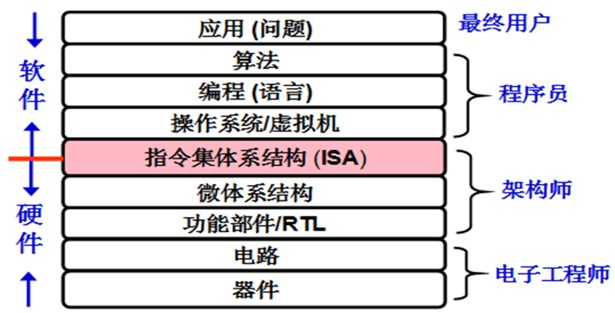# 为什么要学习计算机系统基础

## 一知半解

### 数组求和

int sum(int a[ ], unsigned len) { int i, sum = 0; for (i = 0; i <= len-1; i++) sum += a[i]; return sum; }

`len = 0` 时, 执行 `sum` 函数的for循环时会发生 `Access Violation` , 即"访问违例"异常. 但是, 当参数 `len` 说明为 `int` 型时, `sum` 函数能正确执行, 为什么?

### 整数的平方

`x``y``int` 型, 当 `x = 65535` 时, 则 `y = x*x = -131071` . 为什么?

### 多重定义符号

/*---main.c---*/ #include <stdio.h> int d=100; int x=200; void p1(void); int main() { p1(); printf("d=%d,x=%d\n",d,x); return 0; } /*---p1.c---*/ double d; void p1() { d=1.0; }

### 奇怪的函数返回值

double fun(int i) { volatile double d = {3.14}; volatile long int a; a[i] = 1073741824; /* Possibly out of bounds */ return d; }

`fun` 函数的源码来看, 每次返回的值应该都是 `3.14` , 可是执行 `fun` 函数后发现其结果是:

• `fun(0)``fun(1)``3.14`
• `fun(2)``3.1399998664856`
• `fun(3)``2.00000061035156`
• `fun(4)``3.14` 并会发生 `访问违例` 这是为什么?

### 时间复杂度和功能都相同的程序

void copyij(int src, int dst) { int i,j; for (i = 0; i < 2048; i++) for (j = 0; j < 2048; j++) dst[i][j] = src[i][j]; } void copyji(int src, int dst) { int i,j; for (j = 0; j < 2048; j++) for (i = 0; i < 2048; i++) dst[i][j] = src[i][j]; }

### 网友贴出的一道百度招聘题

#include <stdio.h> int main() { double a = 10; printf("a = %d\n", a); return 0; }

### 整数除法

• 代码段一:
int a = 0x80000000; int b = a / -1; printf("%d\n", b);
• 代码段二:

int a = 0x80000000; int b = -1; int c = a / b; printf("%d\n", c);

代码段一的运行结果为 `-2147483648` ; 而代码段二的运行结果为 `Floating point exception` , 显然, 代码段二运行时被检测到了"溢出"异常. 看似同样功能的程序为什么结果完全不同?

## 站得高, 看得远• 能够对软, 硬件功能进行合理划分
• 能够对系统不同层次进行抽象和封装
• 能够对系统的整体性能进行分析和调优
• 能够对系统各层面的错误进行调试和修正
• 能够根据系统实现机理对用户程序进行准确的性能评估和优化
• 能够根据不同的应用要求合理构建系统框架等

• 对计算机系统整机概念的认识
• 对计算机系统层次结构的深刻理解
• 对高级语言程序, ISA, OS, 编译器, 链接器等之间关系的深入掌握
• 对指令在硬件上执行过程的理解和认识
• 对构成计算机硬件的基本电路特性和设计方法等的基本了解等 从而能够更深刻地理解时空开销和权衡, 抽象和建模, 分而治之, 缓存和局部性, 吞吐率和时延, 并发和并行, 远程过程调用(RPC), 权限和保护等重要的核心概念, 掌握现代计算机系统中最核心的技术和实现方法.

`计算机系统基础` 课程的主要教学目标是培养学生的系统能力, 使其成为一个"高效"程序员, 在程序调试, 性能提升, 程序移植和健壮性等方面成为高手; 建立扎实的计算机系统概念, 为后续的OS, 编译, 体系结构等课程打下坚实基础.

## 实践是检验真理的唯一标准

• (theory, 理论计算机科学) 什么是计算?
• (system, 计算机系统) 什么是计算机?
• (application, 计算机应用) 我们能用计算机做什么?

PhotoShop里面的滤镜功能繁多, 随便点点就能美化图片, 为什么还需要学习图像处理的基本原理?

此页面修订于: 2019-05-03 10:36:40